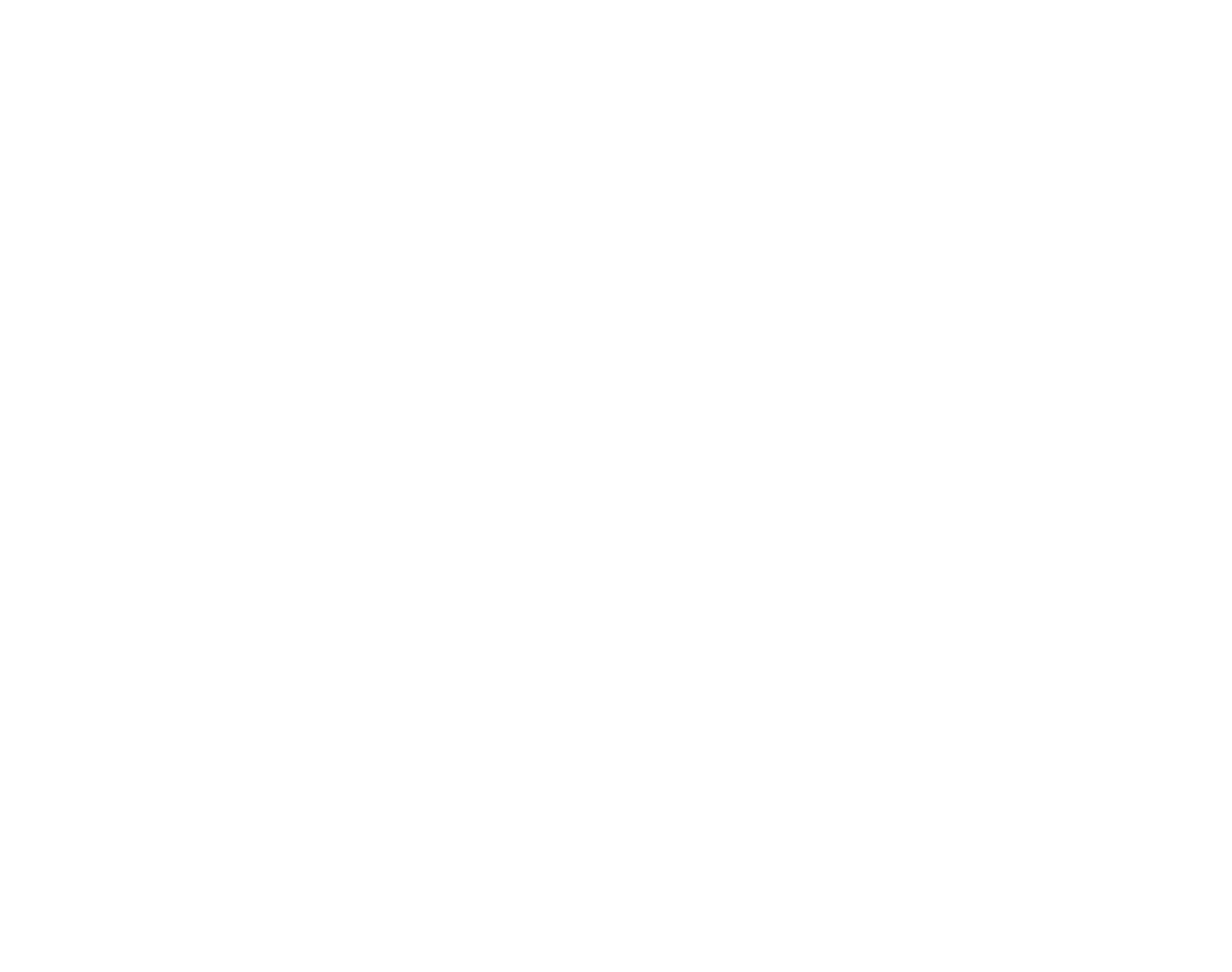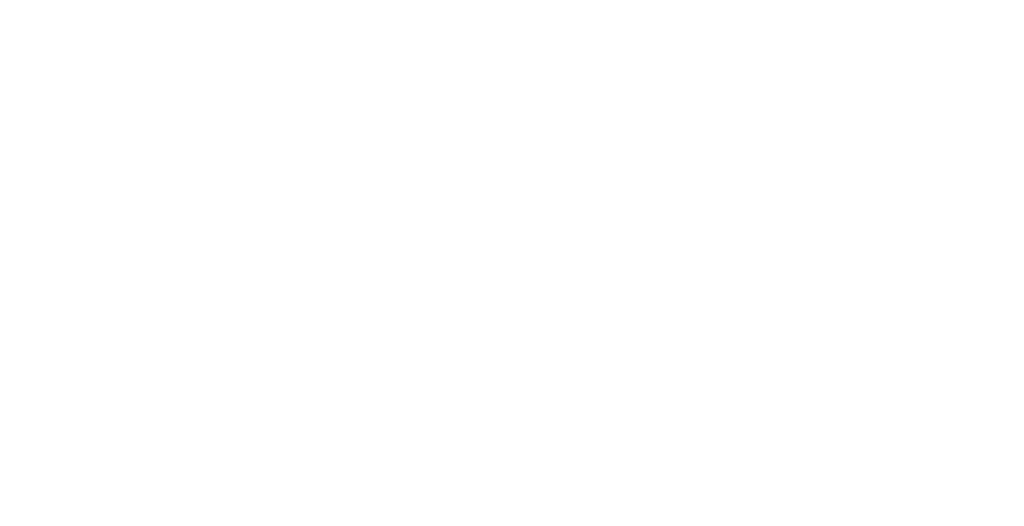EOQ stands for Economic Order Quantity. Economic Order Quantity is calculated by businesses to have a better understanding of what quantity of purchase will be the most cost-effective for them.

Calculating EOQ requires a formula which we will get on later. This formula takes into account all costs attached to storing stocks purchased.

This helps managers avoid shortages and overstocking.

## What is EOQ?

EOQ, Economic Order Quantity, is a way businesses realize the quantity of stock they should order upon purchase.

This is important because purchasing stocks require costs attached to them which add to the cost price of the items. These additional costs are aimed to be minimized by ordering an amount that is the most cost-effective for the business i.e. is the most profitable against the order’s cost.

This is done by the Economic Order Quantity formula taking into account purchasing and storing costs of the inventory. Additionally, the demand for this inventory will also be realized as not to make a business suffer a shortage.

Using the formula, an optimal amount will be derived which minimizes purchasing and storing costs, while also fulfilling the demand of the product in the business.### Example of using EOQ in Purchasing

For example, when a bread baking company wants to purchase Flour, its main raw material ingredient, the manager will tend to calculate the Flour’s Economic Order Quantity of purchase first. The EOQ will tell the manager what amount of quantity should the manager order.

To calculate the amount, the manager will need to know the Flour’s Setup Cost, Holding Cost, and Demand Rate. These terms are further explained below.

Once this information is substituted into the Economic Order Quantity Formula, the manager will derive the optimal quantity of Flour to purchase. This quantity will have minimum setup and holding costs and will be enough for the company’s use as to not create a shortage.

## How To Calculate EOQEOQ is calculated using a fairly easy mathematical formula. This formula uses information relating to other costs and data which the user should know to calculate the Economic Order Quantity.

### EOQ Formula with Example

The formula for deriving Economic Order Quantity is:

### EOQ =√(2SD/H)

I.e. square root of (2 x Setup Costs x Demand) / Holding Costs

### How To Calculate EOQ using the EOQ Formula

To make use of the Economic Order Quantity formula, you will need to understand what the formula comprises.

Ordering(Setup) Cost (S): Ordering cost is how much ordering a purchase costs. This includes shipping and handling costs. Setup cost is per order, for example, if setup cost is \$5, this means each order has the setup cost of \$5.

Demand per year (D): Demand per year tells how much of the product your business demand over a year. This doesn’t necessarily mean how much your product is sold during a year, but how much the product you want to order is used.

For example, if 1000 pieces of bread are sold over a year which used 100 kgs of flour and your company is ordering flour, your demand per year would be 100kgs.

Demand per year can be calculated using historical data.

Holding Cost/Unit (H): Holding Cost/Unit refers to how much it costs to store the particular item in the order. The holding cost of inventory is an important item for using Economic Order Quantity in cost accounting as it tells how much it costs to store a single item of inventory.

### Holding Cost Formula

Holding cost is calculated using a formula. This is:

Holding Cost = (Storage Costs + Employee Salaries + Opportunity Costs + Depreciation Costs) / Total Value of Annual Inventory

### EOQ Example

To demonstrate how EOQ is used, we will view an example of Fenson, a company that manufactures guitars.

Fenson uses wood to manufacture acoustic guitars. The manager of Fenson wants to know the optimal amount of wood she should order. This can be known using EOQ. To enable that, the manager acquires data to be used in the EOQ formula.

To draw up this data, the manager uses historical data of how much wood Fenson demanded last year and is likely to demand this year. With the help of a cost accountant who knows warehouse and purchases department costs, the manager also figures out the Setup Cost per order and the Holding Cost per unit.

This information draws up the following data:

Setup Cost (S) = \$300/order

Demand per year (D) = 15,000 Units/year

Holding Cost (H) = \$1/unit

The manager then substitutes this information into the Economic Order Quantity formula:

√(2x300x15000/1) = 3000

Using the formula, the manager now knows the optimal amount of wood to order would be 3000 units. She draws up a purchase order for exactly this amount.

## Use of EOQ in Inventory Management with ERP Software

EOQ is commonly used in the inventory management of businesses. This is because the Economic Order Quantity shows businesses a cost-effective way of purchasing that doesn’t use up extra costs and also ensures stock is readily available to meet the demand of the business.

An important tool to calculate Economic Order Quantity is ERP, Enterprise Resource Planning.

ERP Software is used by businesses to manage better, streamline operations under a business, and smoothen communications between departments.

One of the main functions of an ERP is inventory management. This includes calculating ratios such as EOQ, Reorder Levels, Maximum Levels etc.

As you read above, in order to calculate EOQ, further information relating to storing and purchasing costs, and annual demand is required. Businesses draw these up from the ERP database their business is already integrated with.

This procedure helps managers to easily calculate important ratios and efficiently help the company’s inventory management.